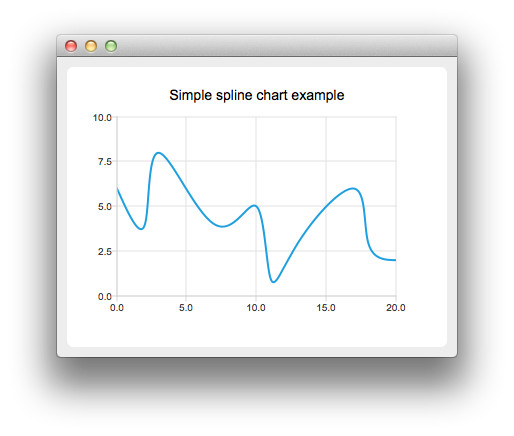# SplineChart Example

The example shows how to create a simple spline chart.#### Running the Example

To run the example from Qt Creator, open the Welcome mode and select the example from Examples. For more information, visit Building and Running an Example.

#### Creating Spline Charts

To create spline charts we need to put our data into QSplineSeries. QSplineSeries automatically calculates spline segment control points that are needed to properly draw the spline.

```QSplineSeries *series = new QSplineSeries();
series->setName("spline");```

Now let's add some data points to the series.

```series->append(0, 6);
series->append(2, 4);
series->append(3, 8);
series->append(7, 4);
series->append(10, 5);
*series << QPointF(11, 1) << QPointF(13, 3) << QPointF(17, 6) << QPointF(18, 3) << QPointF(20, 2);```

The data series has been populated. To display it on a chart we create a QChart object and add the data series to it. We also set the title and the values range on the y-axis, so that our chart's visibility is better.

```QChart *chart = new QChart();
chart->legend()->hide();
chart->setTitle("Simple spline chart example");
chart->createDefaultAxes();
chart->axes(Qt::Vertical).first()->setRange(0, 10);```

Then we create a QChartView object with QChart as a parameter. This way we don't need to create a QGraphicsView scene ourselves. We also set the Antialiasing on to have the rendered lines look nicer.

```QChartView *chartView = new QChartView(chart);
chartView->setRenderHint(QPainter::Antialiasing);```

Finally, we set the QChartView as the windows's central widget.

```QMainWindow window;
window.setCentralWidget(chartView);
window.resize(400, 300);
window.show();```

Example project @ code.qt.io